Solving A Compounding Riddle With Black-Scholes

A few weeks ago I was getting on an airplane armed with a paper and pen, ready to solve the problem in the tweet below. And while I think you will enjoy the approach, the real payoff is going to follow shortly after — I’ll show you how to not only solve it with option theory but expand your understanding of the volatility surface. This is going to be fun. Thinking caps on. Let’s go.

The Question That Launched This Post

From that tweet, you can see the distribution of answers has no real consensus. So don’t let others’ choices affect you. Try to solve the problem yourself. I’ll re-state some focusing details:

• Stock A compounds at 10% per year with no volatility
• Stock B has the same annual expectancy as A but has volatility. Its annual return is binomial — either up 30% or down 10%.
• After 10 years, what’s the chance volatile stock B is higher than A?

You’ll get the most out of this post if you try to solve the problem. Give it a shot. Take note of your gut reactions before you start working through it. In the next section, I will share my gut reaction and solution.

My Approach To The Problem

Gut Reaction

So the first thing I noticed is that this is a “compounding” problem. It’s multiplicative. We are going to be letting our wealth ride and incurring a percent return. We are applying a rate of return to some corpus of wealth that is growing or shrinking. I’m being heavy-handed in identifying that because it stands in contrast to a situation where you earn a return, take profits off the table, and bet again. Or situations, where you bet a fixed amount in a game as opposed to a fraction of your bankroll. This particular poll question is a compounding question, akin to re-investing dividends not spending them. This is the typical context investors reason about when doing “return” math. Your mind should switch into “compounding” mode when you identify these multiplicative situations.

So if this is a compounding problem, and the arithmetic returns for both investments are 10% I immediately know that volatile stock “B” is likely to be lower than stock “A” after 10 years. This is because of the “volatility tax” or what I’ve called the volatility drain. Still, that only conclusively rules out choice #4. Since we could rule that without doing any work and over 2,000 respondents selected it, I know there’s a good reason to write this post!

Showing My Work

Here’s how I reasoned through the problem step-by-step.

Stock A’s Path (10% compounded annually)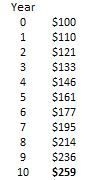Stock B’s Path (up 30% or down 10%)

The fancy term for this is “binomial tree” but it’s an easy concept visually. Let’s start simple and just draw the path for the first 2 years. Up nodes are created by multiplying the stock price by 1.3, down modes are created by multiplying by .90.Inferences

Year 1: 2 cumulative outcomes. Volatile stock B is 50/50 to outperform
Year 2: There are 3 cumulative outcomes. Stock B only outperforms in one of them.

Let’s pause here because while we are mapping the outcome space, we need to recognize that not every one of these outcomes has equal probability.

2 points to keep in mind:

• In a binomial tree, the number of possibilities is 2ᴺ where N is the number of years. This makes sense since each node in the tree has 2 possible outcomes, the tree grows by 2ᴺ.
• However, the number of outcomes is N + 1. So in Year 1, there are 2 possible outcomes. In year 2, 3 possible outcomes.

Probability is the number of ways an outcome can occur divided by the total number of possibilities.

Visually:So by year 2 (N=2), there are 3 outcomes (N+1) and 4 cumulative paths (2ᴺ)

We are moving slowly, but we are getting somewhere.

In year 1, the volatile investment has a 50% chance of winning. The frequency of win paths and lose paths are equal. But what happens in an even year?

There is an odd number of outcomes, with the middle outcome representing the number of winning years and the number of losing years being exactly the same. If the frequency of the wins and losses is the same the volatility tax dominates. If you start with \$100 and make 10% then lose 10% the following year, your cumulative result is a loss.

\$100 x 1.1 x .9 = \$99

Order doesn’t matter.

\$100 x .9 x 1.1 = \$99

In odd years, like year 3, there is a clear winner because the number of wins and losses cannot be the same. Just like a 3-game series.

Solving for year 10

If we extend this logic, it’s clear that year 10 is going to have a big volatility tax embedded in it because of the term that includes stock B having 5 up years and 5 loss years.

N = 10
Outcomes (N+1) = 11 (ie 10 up years, 9 up years, 8 up years…0 up years)
# of paths (2ᴺ) = 1024

We know that 10, 9, 8,7,6 “ups” result in B > A.
We know that 4, 3, 2,1, 0 “ups” result in B < A

The odds of those outcomes are symmetrical. So the question is how often does 5 wins, 5 losses happen? That’s the outcome in which stock A wins because the volatility tax effect is so dominant.

The number of ways to have 5 wins in 10 years is a combination formula for “10 choose 5”:

₁₀C₅ or in Excel =combin(10,5) = 252

So there are 252 out of 1024 total paths in which there are 5 wins and 5 losses. 24.6%

24.6% of the time the volatility tax causes A > B. The remaining paths represent 75.4% of the paths and those have a clear winner that is evenly split between A>B and B>A.

75.4% / 2 = 37.7%

So volatile stock B only outperforms stock A 37.7% of the time despite having the same arithmetic expectancy!

This will surprise nobody who recognized that the geometric mean corresponds to the median of a compounding process. The geometric mean of this investment is not 10% per year but 8.17%. Think of how you compute a CAGR by taking the terminal wealth and raising it to the 1/N power. So if you returned \$2 after 10 years on a \$1 investment your CAGR is 2^(1/10) – 1 = 7.18%. To compute a geometric mean for stock B we invert the math: .9^(1/2) * 1.3^(1/2) -1  = 8.17%. (we’ll come back to this after a few pictures)

The Full Visual

A fun thing to recognize with binomial trees is that the coefficients (ie the number of ways a path can be made that we denoted with the “combination” formula) can be created easily with Pascal’s Triangle. Simply sum the 2 coefficients directly from the line above it.

Coefficients of the binomial expansion (# of ways to form the path)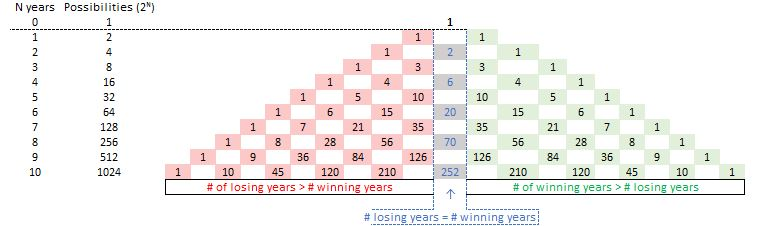Probabilities (# of ways to form each path divided by total paths)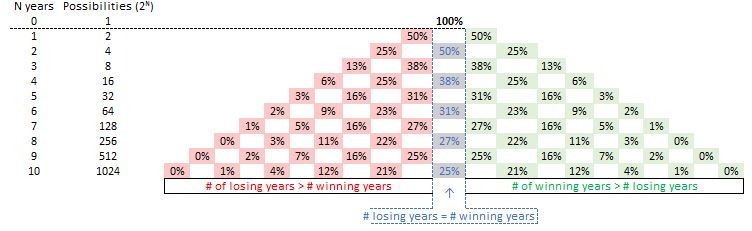Corresponding Price PathsAbove we computed the geometric mean to be 8.17%. If we compounded \$100 at 8.17% for 10 years we end up with \$219 which is the median result that corresponds to 5 up years and 5 down years!

The Problem With This Solution

I solved the 10-year problem by recognizing that, in even years, the volatility tax would cause volatile stock B to lose when the up years and down years occurred equally. (Note that while an equal number of heads and tails is the most likely outcome, it’s still not likely. There’s a 24.6% chance that it happens in 10 trials).

But there’s an issue.

My intuition doesn’t scale for large N. Consider 100 years. Even in the case where B is up 51 times and down 49 times the volatility tax will still cause the cumulative return of B < A. We can use guess-and-test to see how many winning years B needs to have to overcome the tax for N = 100.

N = 100

If we put \$1 into A, it grows at 1.1^100 = \$13,871

If we put \$1 into B and it has 54 winning years and 46 losing years, it will return 1.3^54 * .9^46 = \$11,171. It underperforms A.

If we put \$1 into B and it has 55 winning years and 45 losing years, it will return 1.3^55 * .9^45 = \$16,136. It outperforms A.

So B needs to have 55 “ups”/45 “downs” or about 20% more winning years to overcome the volatility tax. It’s not as simple as it needs to win more times than stock A, like we found for shorter horizons.

We need a better way.

The General Solution Comes From Continuous Compounding: The Gateway To Option Theory

In the question above, we compounded the arithmetic return of 10% annually to get our expectancy for the stocks.

Both stocks’ expected value after 10 years is 100 * 1.1^10 = \$259.37.

Be careful. You don’t want the whole idea of the geometric mean to trip you up. The compounding of volatility does NOT change the expectancy. It changes the distribution of outcomes. This is crucial.

The expectancy is the same, the distribution differs.

If we keep cutting the compounding periods from 1 year to 1 week to 1 minute…we approach continuous compounding. That’s what logreturns are. Continuously compounded returns.

Here’s the key:

Returns conform to a lognormal distribution. You cannot lose more than 100% but you have unlimited upside because of the continuous compounding. Compared to a bell-curve the lognormal distribution is positively skewed. The counterbalance of the positive skew is that the geometric mean or center of mass of the distribution is necessarily lower than the arithmetic expectancy. How much lower? It depends on the volatility because the volatility tax1 pulls the geometric mean down from the arithmetic mean or expectancy. The higher the volatility, the more positively skewed the lognormal or compounded distribution is. The more volatile the asset is in a positively skewed distribution the larger the right tail grows since the left tail is bounded by zero. The counterbalance to the positive skew is that the most likely outcome is the geometric mean.

I’ll pause here for a moment to just hammer home the idea of positive skew:

If stock B doubled 20% of the time and lost 12.5% the remaining 80% of the time its average return would be exactly the same as stock A after 1 year (20% * \$200 + 80% * \$87.5 = \$110). The arithmetic mean is the same. But the most common lived result is that you lose. The more we crank the volatility higher, the more it looks like a lotto ticket with a low probability outcome driving the average return.

Look at the terminal prices for stock B:The arithmetic mean is the same as A, \$259.

The geometric or mean or most likely outcome is only \$219 (again corresponding to the 8.17% geometric return)

The magnitude of that long right tail (\$1,379 is > 1200% total return, while the left tail is a cumulative loss of 65%) is driving that 10% arithmetic return.

Compounding is pulling the typical outcome down as a function of volatility but it’s not changing the overall expectancy.

A Pause To Gather Ourselves

• We now understand that compounded returns are positively skewed.
• We now understand that logreturns are just compounded returns taken continuously as opposed to annually.
• This continuous, logreturn world is the basis of option math.

Black-Scholes

The lognormal distribution underpins the Black-Scholes model used for pricing options.

The mean of a lognormal distribution is the geometric mean. By now we understand that the geometric mean is always lower than the arithmetic mean. So in compounded world we understand that most likely outcome is lower than the arithmetic mean.

Geometric mean  = arithmetic mean – .5 * volatility²

The question we worked on is not continuous compounding but if it were, the geometric mean = 10% – .5 * (.20)² = 8%. Just knowing this was enough to know that most likely B would not outperform A even though they have the same average expectancy.

Let’s revisit the original question, but now we will assume continuous compounding instead of annual compounding. The beauty of this is we can now use Black Scholes to solve it!

Re-framing The Poll As An Options Question

We now switch compounding frequency from annual to continuous so we are officially in Black-Scholes lognormal world.

Expected return (arithmetic mean)

• Annual compounding: \$100 * (1.1)¹⁰ = \$259.37
• Continuous compounding (B-S world): 100*e^(.10 * 10) = \$271.83

Median return (geometric mean)

• Annual compounding: \$100 x 1.0817¹⁰ = \$219.24
• Continuous compounding (B-S world): \$100 * e^(.10 – .5 * .2²) = \$222.55
• remember Geometric mean  = arithmetic mean – .5 * volatility²
• geometric mean < arithmetic mean of course

The original question:

What’s the probability that stock B with its 10% annual return and 20% volatility outperforms stock A with its 10% annual return and no volatility in 10 years?

Asking the question in options language:

What is the probability that a 10-year call option on stock B with a strike price of \$271.83 expires in-the-money?

If you have heard that “delta” is the probability of “expiring in-the-money” then you think we are done. We have all the variables we need to use a Black-Scholes calculator which will spit out a delta. The problem is delta is only approximately the probability of expiring in-the-money. In cases with lots of time to expiry, like this one where the horizon is 10 years, they diverge dramatically. 2

We will need to extract the probability from the Black Scholes equation. Rest assured, we already have all the variables.

Computing The Probability That Stock “B” Expires Above Stock “A”

If we simplify Black-Scholes to a bumper sticker, it is the probability-discounted stock price beyond a fixed strike price. Under the hood of the equation, there must be some notion of a random variable’s probability distribution. In fact, it’s comfortingly simple. The crux of the computation is just calculating z-scores.

I think of a z-score as the “X” coordinate on a graph where the “Y” coordinate is a probability on a distribution. Refresher pic3: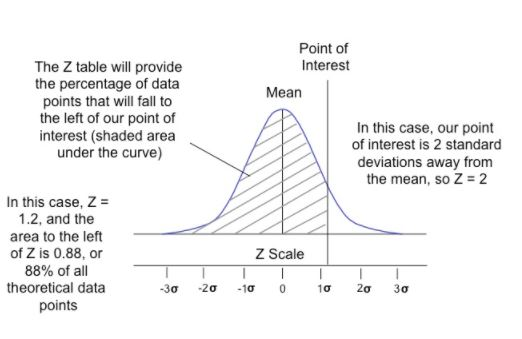Conceptually, a z-score is a distance from a distribution’s mean normalized by its standard deviation. In Black-Scholes world, z-scores are a specified logreturn’s distance from the geometric mean normalized by the stock’s volatility. Same idea as the Gaussian z-scores you have seen before.

Conveniently, logreturns are themselves normally distributed allowing us to use the good ol’ NORM.DIST Excel function to turn those z-scores into probabilities and deltas.

In Black Scholes,

• delta is N(d1)
• probability of expiring in-the-money is N(d2)
• d1 and d2 are z-scores

Here are my calcs4:Boom.

The probability of stock B finishing above stock A (ie the strike or forward price of an a \$100 stock continuously compounded at 10% for 10 years) is…

37.6%!

This is respectably close to the 37.7% we computed using Pascal’s Triangle. The difference is we used the continuous compounding (lognormal) distribution of returns instead of calculating the return outcomes discretely.

The Lognormal Distribution Is A Lesson In How Compounding Influences Returns

I ran all the same inputs through Black Scholes for strikes up to \$750.

• This lets us compute all the straddles and butterflies in Black-Scholes universe (ie what market-makers back in the day called “flat sheets”. That means no additional skew parameters were fit to the model or the model was not fit to the market).
• The flys lets us draw the distribution of prices.

A snippet of the table:I highlighted a few cells of note:

• The 220 strike has a 50% chance of expiring ITM. That makes sense, it’s the geometric mean or arithmetic median.
• The 270 strike is known as At-The-Forward because it corresponds to the forward price of \$271.83 derived from continuously compounding \$100 at 10% per year for 10 years (ie Seʳᵗ). If 10% were a risk-free rate this would be treated like the 10 year ATM price in practice. Notice it has a 63% delta. This suprises people new to options but for veterans this is expected (assuming you are running a model without spot-vol correlation).
• You have to go to the \$330 strike to find the 50% delta option! If you need to review why see Lessons From The .50 Delta Option.

This below summary picture adds one more lesson:

The cheapest straddle (and therefore most expensive butterfly) occurs at the modal return, about \$150. If the stock increased from \$100 to \$150, you’re CAGR would be 4.1%. This is the single most likely event despite the fact that it’s below the median AND has a point probability of only 1.7%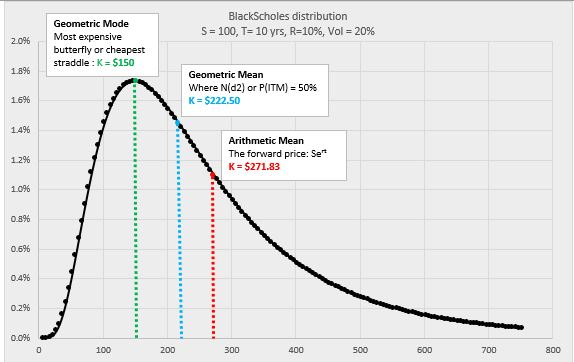Speaking of Skew

Vanilla Black-Scholes option theory is a handy framework for understanding the otherwise unintuitive hand of compounding. The lognormal distribution is the distribution that corresponds to continuously compounded returns. However, it is important to recognize that nobody actually believes this distribution describes any individual investment. A biotech stock might be bimodally distributed, contingent on an FDA approval. If you price SPX index options with positively skewed model like this you will not last long.

A positively skewed distribution says “on average I’ll make X because sometimes I’ll make multiples of X but most of the time, my lived experience is I’ll make less than X”.

In reality, the market imputes negative skew on the SPX options market. This shifts the peak to the right, shortens the right tail, and fattens the left tail. That implied skew says “on average I make X, I often make more than X, because occasionally I get annihilated”.

It often puzzles beginning traders that adding “put skew” to a market, which feels like a “negative” sentiment, raises the value of call spreads. But that actually makes sense. A call spread is a simple over/under bet that reduces to the odds of some outcome happening. If the spot price is unchanged, and the puts become more expensive because the left tail is getting fatter, then it means the asset must be more likely to appreciate to counterbalance those 2 conditions. So of course the call spreads must be worth more.

Final Wrap

Compounding is a topic that gives beginners and even experienced professionals difficulty. By presenting the solution to the question from a discrete binomial angle and a continuous Black-Scholes angle, I hope it soldified or even furthered your appreciation for how compounding works.

My stretch goal was to advance your understanding of option theory. While it overlaps with many of my other option theory posts, if it led to even any small additional insight, I figure it’s worth it. I enjoyed sensing that the question could be solved using options and then proving it out.

I want to thank @10kdiver for the work he puts out consistently and the conversation we had over Twitter DM regarding his question. If you are trying to learn basic and intermediate level financial numeracy his collection of threads is unparalled. Work I aspire to. Check them out here: https://10kdiver.com/twitter-threads/

Remember, my first solution (Pascal’s Triangle) only worked for relatively small N. It was not a general solution. The Black-Scholes solution is a general one but required changing “compounded annually” to “compounded continuously”. 10kdiver provided the general solution, using logs (so also moving into continuous compounding) but did not require discussion of option theory.

I’ll leave you with that:

• Path: How Compounding Alters Return Distributions (Link)

This post shows how return distributions built from compounding depend on the ratio of trend vs chop.

• The difficulty with shorting and inverse positions (Link)

The reason shorting and inverse positions are problematic is intimately tied to compounding math.

1. Oscillating between .9 and 1.1 leaves you below 1. Now imagine a more volatile investment: .5 * 1.5 = .75. If you gain and then lose 50%, you have cumulatively lost 25%!
2. In Black Scholes:

• The term for delta is N(d1).
• The term for the probability of finishing in the money is N(d2).

What’s the relationship between d2 and d1?

• d2 = d1 – σ√t

The higher the volatility or the more time there is to expiry, the more delta and probability diverge!

Delta and probability are only similar when an option is near expiration or when it’s vol is “low”. For a fuller discussion what it means for delta to be a hedge ratio see:

Lessons From The .50 Delta Option